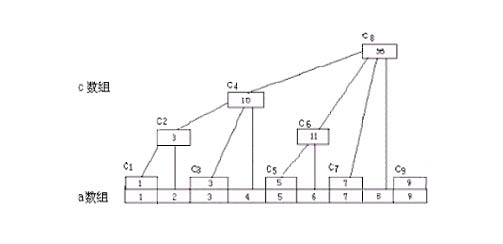# 树状数组

1、例如： 元数据 a[] , 树状数组c[]  c[n] 表示的段是 c[n] = a[n-2^k +1 ] + a[n-2^k] + ......+ a[n]
注意 k 表示的是二进制 下末尾 0 的个数.....
要思考的问题是如何 根据 n  求出2^ k 的值

如图所示：c = a  ;
c = a + a ;
c = a ;
c = c + c + a = a +a +a + a ;
c = a;
c = a + a  ;
。。。。。。
1)  上面继续想问题 k 表示的是  n 在二进制下0 的个数   例如 6 （110） 结果是 1  输出 2^k  = 2 用什么方法来得到呢？
2^k = n and (n xor (n-1))
这个公式不难推导  先验证一下正确性  110 and(110 xor 101) = 110 and 011 = 010 = 2D

int lowbit(int x)
{//函数功能主要是根据n 求2^k 方便动态维护和查询更新
return x&(-x);
}

2) 要想的问题就是 更新维护操作了(下面讨论一维的)

void update1(int pos, int w)
{
//这里讨论的是一维的
/*也可以写成
while(pos<=n)
{
c[pos] += w;
pos+= lowbit(pos);
}
*/
int i;
for(i = pos;i <= n ; i+=lowbit(i))
{
c[pos] += w;
}
}

void update2(int x, int y,int w)
{//这里是二维的树状数组的更新方便查询和维护
int i , j;
for(i = x ; i <= n ; i +=lobit(i))
{
for(j = y ; j <= n ;j+=lowbit(j))
{
c[i][j] += w;
}
}
}

3）下面要讨论的就是求和操作了 其实树状数组的产生也是为了降低复杂度而生成的 求和操作也可以说成是查询

例如: 上面 6 110 －lowbit(6) 即 110 - 010 得到100  6-2 = 4
对于N 其实最多有logN个1   这个很容易理解　　因此查询效率可以降低到log(N)

int sum（int x, int y)
{
/*也可以写成
int sum = 0;
int tmp;
while( x > 0 )
{
tmp = y;
while(tmp >0)
{
sum += c[i][tmp] ;
tmp -= lowbit(tmp);
}
i -= lowbit(i);
}
return sum;
*/
int sum = 0;
int i , j;
for(i= x ; i > 0 ; i -=lowbit(i))
{
for(j = y ; j > 0 ; j -= lowbit(j))
{
sum += c[i][j];
}
}
return sum;
}

int sum1(int en)
{//这个主要是一维树状数组的求和问题了
/*也可以写成
int sum = 0;
int tmp = en;
while(tmp > 0)
{
sum += c[tmp] ;
tmp -= lowbit(tmp);
}
return sum ;
*/
int j ;
int sum = 0
for(j = en ; j > 0 ;j-= lowbit(j))
{
sum  += c[j];
}
return sum;
}

树状数组能有效的改善求和问题的复杂度，但是也有他一定的局限性。。

2) 对于不能有效的利用 sum(a, b) = sum(1, b) - sum(1, a-1) 进行操作的也是不可以的

更行操作 每次都市lowbit(N) 一下因此复杂度可以说是O(log(n))
求和操作或者说是查询操作复杂度也是　O(log(n))
以上每次都市减掉或者加上最低位1  复杂度可以降低到 logn

#include<iostream>
using namespace std;

int lowbit(int x)
{//函数功能主要是根据n 求2^k 方便动态维护和查询更新
return x&(-x);
}
void update1(int pos, int w)
{
//这里讨论的是一维的
/*也可以写成
while(pos>0)
{
c[pos] += num;
pos-= lowbit(pos);
}
*/
int i;
for(i = pos;i > 0 ; i-=lowbit(i))
{
c[pos] += w;
}
}
void update2(int x, int y,int w)
{//这里是二维的树状数组的更新方便查询和维护
int i , j;
for(i = x ; i <= n ; i +=lobit(i))
{
for(j = y ; j <= n ;j+=lowbit(j))
{
c[i][j] += w;
}
}
}
int sum1(int en)
{//这个主要是一维树状数组的求和问题了
/*也可以写成
int sum = 0;
int tmp = en;
while(tmp > 0)
{
sum += c[tmp] ;
tmp -= lowbit(tmp);
}
return sum ;
*/
int j ;
int sum = 0
for(j = en ; j > 0 ;j-= lowbit(j))
{
sum  += c[j];
}
return sum;
}
//下面的代码查询a ~ a[i][j] 之间的和
int sum2（int x, int y)
{
/*也可以写成
int sum = 0;
int tmp;
while( x > 0 )
{
tmp = y;
while(tmp >0)
{
sum += c[i][tmp] ;
tmp -= lowbit(tmp);
}
i -= lowbit(i);
}
return sum;
*/
int sum = 0;
int i , j;
for(i= x ; i > 0 ; i -=lowbit(i))
{
for(j = y ; j > 0 ; j -= lowbit(j))
{
sum += c[i][j];
}
}
return sum;
}
int main()
{

return 0;
}


10-23
09-1607-23
01-03
11-24
01-06
08-20
05-03
06-254万+
01-254万+
06-11
09-03
04-01
05-14
02-18
08-16
©️2020 CSDN 皮肤主题: Age of Ai 设计师:meimeiellie点击重新获取扫码支付1.余额是钱包充值的虚拟货币，按照1:1的比例进行支付金额的抵扣。
2.余额无法直接购买下载，可以购买VIP、C币套餐、付费专栏及课程。余额充值